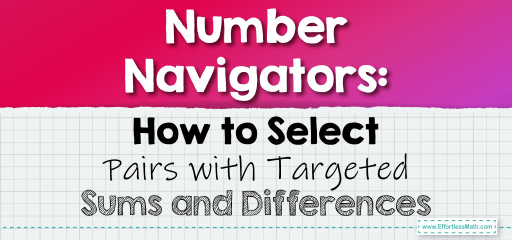# Number Navigators: How to Select Pairs with Targeted Sums and Differences

Sometimes, we're faced with challenges where we need to find pairs of numbers that either sum up or have a specific difference. This skill is essential for various mathematical and real-world scenarios. Let's dive into the methods to pick such number pairs.

## Picking Numbers with a Specific Difference or Sum### Example 1:

Pick two numbers that sum up to $$15$$.

Solution Process:

There are multiple pairs that can sum up to $$15$$, such as:

1. $$7 + 8$$

2. $$6 + 9$$

3. $$5 + 10$$

… and so on.

One possible pair is $$7$$ and $$8$$.

The Absolute Best Book for 5th Grade Students

### Example 2:

Pick two numbers that have a difference of $$5$$.

Solution Process:

Again, multiple pairs can have a difference of $$5$$, such as:

1. $$10 – 5$$

2. $$15 – 10$$

3. $$20 – 15$$

… and so on.

One possible pair is $$10$$ and $$5$$.

Picking numbers with a specific sum or difference is a foundational skill in mathematics. It’s crucial for problem-solving, especially in algebra and arithmetic. By understanding the relationship between numbers and their operations, you can quickly identify pairs that meet the criteria. This skill is handy for various applications, from splitting bills to budgeting and planning. Keep practicing to enhance your ability to swiftly pick the right number pairs!

### Practice Questions:

1. Find two numbers that sum up to $$20$$.

2. Pick two numbers with a difference of $$7$$.

3. Choose a pair of numbers that sum up to $$30$$.

4. Identify two numbers with a difference of $$3$$.

5. Select two numbers that, when added, give $$50$$.

A Perfect Book for Grade 5 Math Word Problems!

1. $$10$$ and $$10$$, $$11$$ and $$9$$, $$12$$ and $$8$$, etc.

2. $$10$$ and $$3$$, $$14$$ and $$7$$, $$20$$ and $$13$$, etc.

3. $$15$$ and $$15$$, $$20$$ and $$10$$, $$25$$ and $$5$$, etc.

4. $$7$$ and $$4$$, $$10$$ and $$7$$, $$13$$ and $$10$$, etc.

5. $$25$$ and $$25$$, $$30$$ and $$20$$, $$35$$ and $$15$$, etc.

The Best Math Books for Elementary Students

### What people say about "Number Navigators: How to Select Pairs with Targeted Sums and Differences - Effortless Math: We Help Students Learn to LOVE Mathematics"?

No one replied yet.

X
51% OFF

Limited time only!

Save Over 51%

SAVE $15 It was$29.99 now it is \$14.99